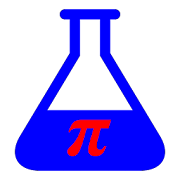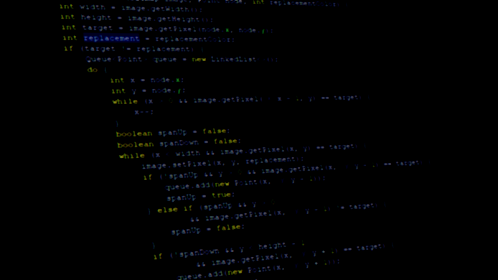# Math LaboratoryEveryone
5
You can solve most of your math problems, these are the supported actions:
- Matrix operations
- invert
- transpose
- Vector operarions
- cross product
- dot product
- Calculus functions
- sums an expression from lower to upper limit
- calculates the product of an expression from lower to upper limit
- gets the derivative
- compute integral of the expression
- compute the definite integral of the expression
- Algebra functions
- divides 2 polynomial
- factor an expression
- gets the greatest common divisor of 2 polynomials
- finds the roots of a univariate polynomial
- get the coefficients of a polynomial
- Solve an equation
- Solves a system of linear equations
- Calculate the Laplace transform of an expression
- Statistical functions: mean, mode, median, zscore, sample variance, population variance, sample standard deviation, population standard deviation
- Use custom variables in your calculus
- Define your own functions to evaluate
- Plot 2D equations
Collapse

Review Policy
2.4
5 total
5
4
3
2
1

## What's New

- Some interface improvements
- If you click in a operation, it gets copied to the input
- Examples are clickable
Collapse

Updated
January 15, 2018
Size
4.4M
Installs
500+
Current Version
0.2.1
Requires Android
4.0.3 and up
Content Rating
Everyone
Permissions
Offered By
Apps by Pablo
Developer
Conchidos nº8 C.P. 36152 Pontevedra, Spain